## 5.403. tree

Origin

N. Beldiceanu

Constraint

$\mathrm{𝚝𝚛𝚎𝚎}\left(\mathrm{𝙽𝚃𝚁𝙴𝙴𝚂},\mathrm{𝙽𝙾𝙳𝙴𝚂}\right)$

Arguments
 $\mathrm{𝙽𝚃𝚁𝙴𝙴𝚂}$ $\mathrm{𝚍𝚟𝚊𝚛}$ $\mathrm{𝙽𝙾𝙳𝙴𝚂}$ $\mathrm{𝚌𝚘𝚕𝚕𝚎𝚌𝚝𝚒𝚘𝚗}\left(\mathrm{𝚒𝚗𝚍𝚎𝚡}-\mathrm{𝚒𝚗𝚝},\mathrm{𝚜𝚞𝚌𝚌}-\mathrm{𝚍𝚟𝚊𝚛}\right)$
Restrictions
 $\mathrm{𝙽𝚃𝚁𝙴𝙴𝚂}\ge 1$ $\mathrm{𝙽𝚃𝚁𝙴𝙴𝚂}\le |\mathrm{𝙽𝙾𝙳𝙴𝚂}|$ $\mathrm{𝚛𝚎𝚚𝚞𝚒𝚛𝚎𝚍}$$\left(\mathrm{𝙽𝙾𝙳𝙴𝚂},\left[\mathrm{𝚒𝚗𝚍𝚎𝚡},\mathrm{𝚜𝚞𝚌𝚌}\right]\right)$ $\mathrm{𝙽𝙾𝙳𝙴𝚂}.\mathrm{𝚒𝚗𝚍𝚎𝚡}\ge 1$ $\mathrm{𝙽𝙾𝙳𝙴𝚂}.\mathrm{𝚒𝚗𝚍𝚎𝚡}\le |\mathrm{𝙽𝙾𝙳𝙴𝚂}|$ $\mathrm{𝚍𝚒𝚜𝚝𝚒𝚗𝚌𝚝}$$\left(\mathrm{𝙽𝙾𝙳𝙴𝚂},\mathrm{𝚒𝚗𝚍𝚎𝚡}\right)$ $\mathrm{𝙽𝙾𝙳𝙴𝚂}.\mathrm{𝚜𝚞𝚌𝚌}\ge 1$ $\mathrm{𝙽𝙾𝙳𝙴𝚂}.\mathrm{𝚜𝚞𝚌𝚌}\le |\mathrm{𝙽𝙾𝙳𝙴𝚂}|$
Purpose

Given a digraph $G$ described by the $\mathrm{𝙽𝙾𝙳𝙴𝚂}$ collection, cover $G$ by a set of $\mathrm{𝙽𝚃𝚁𝙴𝙴𝚂}$ trees in such a way that each vertex of $G$ belongs to one distinct tree. The edges of the trees are directed from their leaves to their respective roots.

Example
 $\left(\begin{array}{c}2,〈\begin{array}{cc}\mathrm{𝚒𝚗𝚍𝚎𝚡}-1\hfill & \mathrm{𝚜𝚞𝚌𝚌}-1,\hfill \\ \mathrm{𝚒𝚗𝚍𝚎𝚡}-2\hfill & \mathrm{𝚜𝚞𝚌𝚌}-5,\hfill \\ \mathrm{𝚒𝚗𝚍𝚎𝚡}-3\hfill & \mathrm{𝚜𝚞𝚌𝚌}-5,\hfill \\ \mathrm{𝚒𝚗𝚍𝚎𝚡}-4\hfill & \mathrm{𝚜𝚞𝚌𝚌}-7,\hfill \\ \mathrm{𝚒𝚗𝚍𝚎𝚡}-5\hfill & \mathrm{𝚜𝚞𝚌𝚌}-1,\hfill \\ \mathrm{𝚒𝚗𝚍𝚎𝚡}-6\hfill & \mathrm{𝚜𝚞𝚌𝚌}-1,\hfill \\ \mathrm{𝚒𝚗𝚍𝚎𝚡}-7\hfill & \mathrm{𝚜𝚞𝚌𝚌}-7,\hfill \\ \mathrm{𝚒𝚗𝚍𝚎𝚡}-8\hfill & \mathrm{𝚜𝚞𝚌𝚌}-5\hfill \end{array}〉\hfill \end{array}\right)$ $\left(\begin{array}{c}8,〈\begin{array}{cc}\mathrm{𝚒𝚗𝚍𝚎𝚡}-1\hfill & \mathrm{𝚜𝚞𝚌𝚌}-1,\hfill \\ \mathrm{𝚒𝚗𝚍𝚎𝚡}-2\hfill & \mathrm{𝚜𝚞𝚌𝚌}-2,\hfill \\ \mathrm{𝚒𝚗𝚍𝚎𝚡}-3\hfill & \mathrm{𝚜𝚞𝚌𝚌}-3,\hfill \\ \mathrm{𝚒𝚗𝚍𝚎𝚡}-4\hfill & \mathrm{𝚜𝚞𝚌𝚌}-4,\hfill \\ \mathrm{𝚒𝚗𝚍𝚎𝚡}-5\hfill & \mathrm{𝚜𝚞𝚌𝚌}-5,\hfill \\ \mathrm{𝚒𝚗𝚍𝚎𝚡}-6\hfill & \mathrm{𝚜𝚞𝚌𝚌}-6,\hfill \\ \mathrm{𝚒𝚗𝚍𝚎𝚡}-7\hfill & \mathrm{𝚜𝚞𝚌𝚌}-7,\hfill \\ \mathrm{𝚒𝚗𝚍𝚎𝚡}-8\hfill & \mathrm{𝚜𝚞𝚌𝚌}-8\hfill \end{array}〉\hfill \end{array}\right)$ $\left(\begin{array}{c}7,〈\begin{array}{cc}\mathrm{𝚒𝚗𝚍𝚎𝚡}-1\hfill & \mathrm{𝚜𝚞𝚌𝚌}-6,\hfill \\ \mathrm{𝚒𝚗𝚍𝚎𝚡}-2\hfill & \mathrm{𝚜𝚞𝚌𝚌}-2,\hfill \\ \mathrm{𝚒𝚗𝚍𝚎𝚡}-3\hfill & \mathrm{𝚜𝚞𝚌𝚌}-3,\hfill \\ \mathrm{𝚒𝚗𝚍𝚎𝚡}-4\hfill & \mathrm{𝚜𝚞𝚌𝚌}-4,\hfill \\ \mathrm{𝚒𝚗𝚍𝚎𝚡}-5\hfill & \mathrm{𝚜𝚞𝚌𝚌}-5,\hfill \\ \mathrm{𝚒𝚗𝚍𝚎𝚡}-6\hfill & \mathrm{𝚜𝚞𝚌𝚌}-6,\hfill \\ \mathrm{𝚒𝚗𝚍𝚎𝚡}-7\hfill & \mathrm{𝚜𝚞𝚌𝚌}-7,\hfill \\ \mathrm{𝚒𝚗𝚍𝚎𝚡}-8\hfill & \mathrm{𝚜𝚞𝚌𝚌}-8\hfill \end{array}〉\hfill \end{array}\right)$

The first $\mathrm{𝚝𝚛𝚎𝚎}$ constraint holds since the graph associated with the items of the $\mathrm{𝙽𝙾𝙳𝙴𝚂}$ collection corresponds to two trees (i.e., $\mathrm{𝙽𝚃𝚁𝙴𝙴𝚂}=2$): each tree respectively involves the vertices $\left\{1,2,3,5,6,8\right\}$ and $\left\{4,7\right\}$. They are depicted by Figure 5.403.1.

##### Figure 5.403.1. The two trees corresponding to the first example of the Example slot; each vertex contains the information $\mathrm{𝚒𝚗𝚍𝚎𝚡}|\mathrm{𝚜𝚞𝚌𝚌}$ where $\mathrm{𝚜𝚞𝚌𝚌}$ is the index of its father in the tree (by convention the father of the root is the root itself).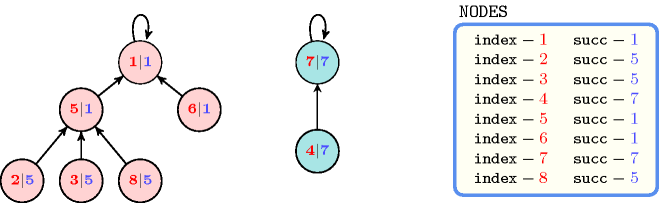All solutions

Figure 5.403.2 gives all solutions to the following non ground instance of the $\mathrm{𝚝𝚛𝚎𝚎}$ constraint: $\mathrm{𝙽𝚃𝚁𝙴𝙴𝚂}\in \left[3,4\right]$, ${S}_{1}\in \left[1,2\right]$, ${S}_{2}\in \left[1,3\right]$, ${S}_{3}\in \left[1,4\right]$, ${S}_{4}\in \left[2,4\right]$, $\mathrm{𝚝𝚛𝚎𝚎}$$\left(\mathrm{𝙽𝚃𝚁𝙴𝙴𝚂},〈1{S}_{1},2{S}_{2},3{S}_{3},4{S}_{4}〉\right)$.

##### Figure 5.403.2. All solutions corresponding to the non ground example of the $\mathrm{𝚝𝚛𝚎𝚎}$ constraint of the All solutions slot (the $\mathrm{𝚒𝚗𝚍𝚎𝚡}$ attribute is displayed as indices of the $\mathrm{𝚜𝚞𝚌𝚌}$ attribute)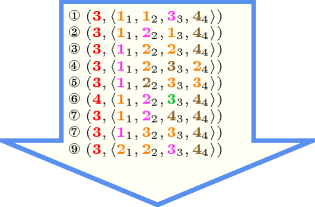Typical
 $\mathrm{𝙽𝚃𝚁𝙴𝙴𝚂}<|\mathrm{𝙽𝙾𝙳𝙴𝚂}|$ $|\mathrm{𝙽𝙾𝙳𝙴𝚂}|>2$
Symmetry

Items of $\mathrm{𝙽𝙾𝙳𝙴𝚂}$ are permutable.

Arg. properties

Functional dependency: $\mathrm{𝙽𝚃𝚁𝙴𝙴𝚂}$ determined by $\mathrm{𝙽𝙾𝙳𝙴𝚂}$.

Remark

Given a complete digraph of $n$ vertices as well as an unrestricted number of trees $\mathrm{𝙽𝚃𝚁𝙴𝙴𝚂}$, the total number of solutions to the corresponding $\mathrm{𝚝𝚛𝚎𝚎}$ constraint corresponds to the sequence A000272 of the On-Line Encyclopaedia of Integer Sequences [Sloane10].

Extension of the $\mathrm{𝚝𝚛𝚎𝚎}$ constraint to the minimum spanning tree constraint is described in [DoomsKatriel06], [Regin08], [ReginRousseauRueherHoeve10].

Algorithm

An arc-consistency filtering algorithm for the $\mathrm{𝚝𝚛𝚎𝚎}$ constraint is described in [BeldiceanuFlenerLorca05]. This algorithm is based on a necessary and sufficient condition that we now depict.

To any $\mathrm{𝚝𝚛𝚎𝚎}$ constraint we associate the digraph $G=\left(V,E\right)$, where:

• To each item $\mathrm{𝙽𝙾𝙳𝙴𝚂}\left[i\right]$ of the $\mathrm{𝙽𝙾𝙳𝙴𝚂}$ collection corresponds a vertex ${v}_{i}$ of $G$.

• For every pair of items $\left(\mathrm{𝙽𝙾𝙳𝙴𝚂}\left[i\right],\mathrm{𝙽𝙾𝙳𝙴𝚂}\left[j\right]\right)$ of the $\mathrm{𝙽𝙾𝙳𝙴𝚂}$ collection, where $i$ and $j$ are not necessarily distinct, there is an arc from ${v}_{i}$ to ${v}_{j}$ in $E$ if and only if $j$ is a potential value of $\mathrm{𝙽𝙾𝙳𝙴𝚂}\left[i\right].\mathrm{𝚜𝚞𝚌𝚌}$.

A strongly connected component $C$ of $G$ is called a sink component if all the successors of all vertices of $C$ belong to $C$. Let $\mathrm{𝙼𝙸𝙽𝚃𝚁𝙴𝙴𝚂}$ and $\mathrm{𝙼𝙰𝚇𝚃𝚁𝙴𝙴𝚂}$ respectively denote the number of sink components of $G$ and the number of vertices of $G$ with a loop.

The $\mathrm{𝚝𝚛𝚎𝚎}$ constraint has a solution if and only if:

• Each sink component of $G$ contains at least one vertex with a loop,

• The domain of $\mathrm{𝙽𝚃𝚁𝙴𝙴𝚂}$ has at least one value within interval $\left[\mathrm{𝙼𝙸𝙽𝚃𝚁𝙴𝙴𝚂},\mathrm{𝙼𝙰𝚇𝚃𝚁𝙴𝙴𝚂}\right]$.

Inspired by the idea of using dominators used in [ItalianoLauraSantaroni10] for getting a linear time algorithm for computing strong articulation points of a digraph $G$, the worst case complexity of the algorithm proposed in [BeldiceanuFlenerLorca05] was also enhanced in a similar way by J.-G. Fages and X. Lorca [FagesLorca11].

Reformulation

The $\mathrm{𝚝𝚛𝚎𝚎}$ constraint can be expressed in term of (1) a set of ${|\mathrm{𝙽𝙾𝙳𝙴𝚂}|}^{2}$ reified constraints for avoiding circuit between more than one node and of (2) $|\mathrm{𝙽𝙾𝙳𝙴𝚂}|$ reified constraints and of one sum constraint for counting the trees:

1. For each vertex $\mathrm{𝙽𝙾𝙳𝙴𝚂}\left[i\right]$ $\left(i\in \left[1,|\mathrm{𝙽𝙾𝙳𝙴𝚂}|\right]\right)$ of the $\mathrm{𝙽𝙾𝙳𝙴𝚂}$ collection we create a variable ${R}_{i}$ that takes its value within interval $\left[1,|\mathrm{𝙽𝙾𝙳𝙴𝚂}|\right]$. This variable represents the rank of vertex $\mathrm{𝙽𝙾𝙳𝙴𝚂}\left[i\right]$ within a solution. It is used to prevent the creation of circuit involving more than one vertex as explained now. For each pair of vertices $\mathrm{𝙽𝙾𝙳𝙴𝚂}\left[i\right],\mathrm{𝙽𝙾𝙳𝙴𝚂}\left[j\right]$ $\left(i,j\in \left[1,|\mathrm{𝙽𝙾𝙳𝙴𝚂}|\right]\right)$ of the $\mathrm{𝙽𝙾𝙳𝙴𝚂}$ collection we create a reified constraint of the form $\mathrm{𝙽𝙾𝙳𝙴𝚂}\left[i\right].\mathrm{𝚜𝚞𝚌𝚌}=\mathrm{𝙽𝙾𝙳𝙴𝚂}\left[j\right].\mathrm{𝚒𝚗𝚍𝚎𝚡}\wedge i\ne j⇒{R}_{i}<{R}_{j}$. The purpose of this constraint is to express the fact that, if there is an arc from vertex $\mathrm{𝙽𝙾𝙳𝙴𝚂}\left[i\right]$ to another vertex $\mathrm{𝙽𝙾𝙳𝙴𝚂}\left[j\right]$, then ${R}_{i}$ should be strictly less than ${R}_{j}$.

2. For each vertex $\mathrm{𝙽𝙾𝙳𝙴𝚂}\left[i\right]$ $\left(i\in \left[1,|\mathrm{𝙽𝙾𝙳𝙴𝚂}|\right]\right)$ of the $\mathrm{𝙽𝙾𝙳𝙴𝚂}$ collection we create a 0-1 variable ${B}_{i}$ and state the following reified constraint $\mathrm{𝙽𝙾𝙳𝙴𝚂}\left[i\right].\mathrm{𝚜𝚞𝚌𝚌}=\mathrm{𝙽𝙾𝙳𝙴𝚂}\left[i\right].\mathrm{𝚒𝚗𝚍𝚎𝚡}⇔{B}_{i}$ in order to force variable ${B}_{i}$ to be set to value 1 if and only if there is a loop on vertex $\mathrm{𝙽𝙾𝙳𝙴𝚂}\left[i\right]$. Finally we create a constraint $\mathrm{𝙽𝚃𝚁𝙴𝙴𝚂}={B}_{1}+{B}_{2}+\cdots +{B}_{|\mathrm{𝙽𝙾𝙳𝙴𝚂}|}$ for stating the fact that the number of trees is equal to the number of loops of the graph.

Counting
 Length ($n$) 2 3 4 5 6 7 8 Solutions 3 16 125 1296 16807 262144 4782969

Number of solutions for $\mathrm{𝚝𝚛𝚎𝚎}$: domains $0..n$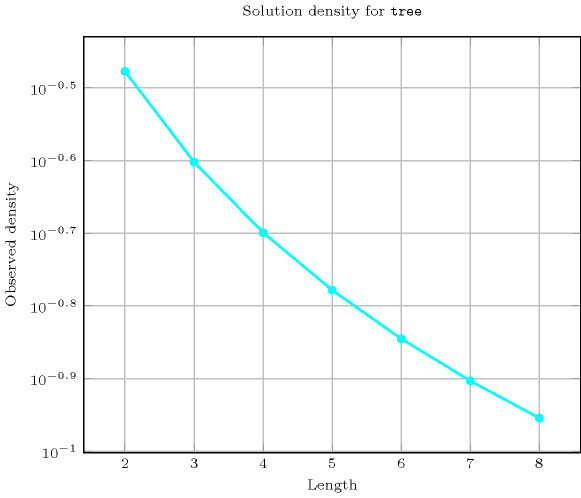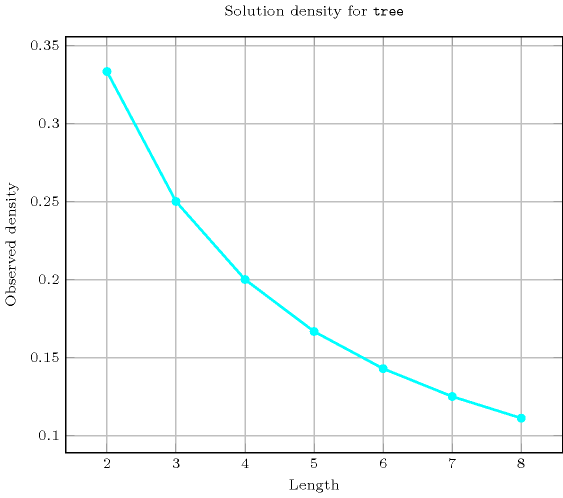Length ($n$)2345678
Total3161251296168072621444782969
 Parameter value

1296462577761176492097152
2164850064801008421835008
3-112150216036015688128
4--1203606860143360
5---13073517920
6----1421344
7-----156
8------1

Solution count for $\mathrm{𝚝𝚛𝚎𝚎}$: domains $0..n$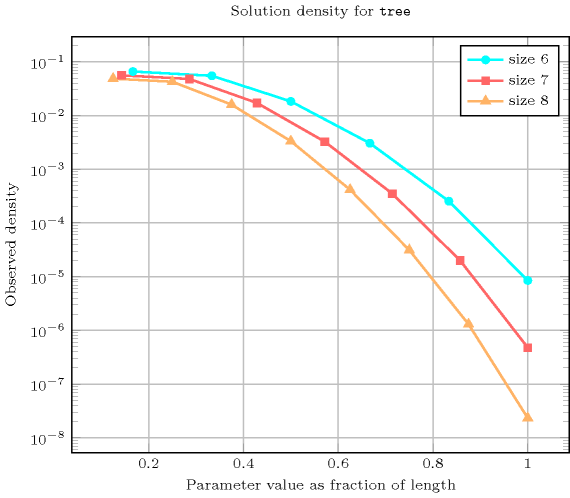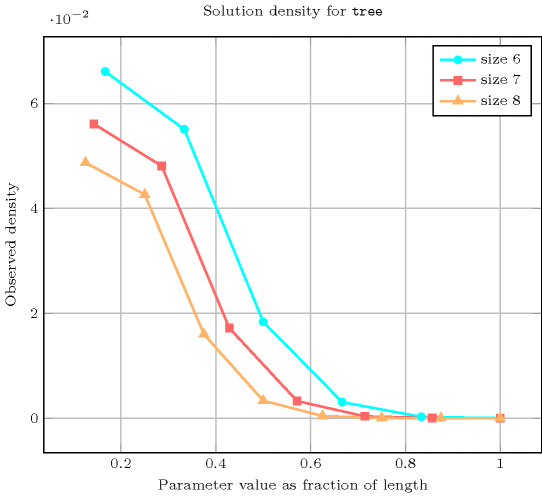Systems

tree in Choco.

related: $\mathrm{𝚋𝚊𝚕𝚊𝚗𝚌𝚎}_\mathrm{𝚝𝚛𝚎𝚎}$ (counting number of trees versus controlling how balanced the trees are), $\mathrm{𝚐𝚕𝚘𝚋𝚊𝚕}_\mathrm{𝚌𝚊𝚛𝚍𝚒𝚗𝚊𝚕𝚒𝚝𝚢}_\mathrm{𝚕𝚘𝚠}_\mathrm{𝚞𝚙}_\mathrm{𝚗𝚘}_\mathrm{𝚕𝚘𝚘𝚙}$, $\mathrm{𝚐𝚕𝚘𝚋𝚊𝚕}_\mathrm{𝚌𝚊𝚛𝚍𝚒𝚗𝚊𝚕𝚒𝚝𝚢}_\mathrm{𝚗𝚘}_\mathrm{𝚕𝚘𝚘𝚙}$ (can be used for restricting number of children since discard loops associated with tree roots).

specialisation: $\mathrm{𝚋𝚒𝚗𝚊𝚛𝚢}_\mathrm{𝚝𝚛𝚎𝚎}$ (no limit on the number of children replaced by at most two children), $\mathrm{𝚙𝚊𝚝𝚑}$ (no limit on the number of children replaced by at most one child).

Keywords
Arc input(s)

$\mathrm{𝙽𝙾𝙳𝙴𝚂}$

Arc generator
$\mathrm{𝐶𝐿𝐼𝑄𝑈𝐸}$$↦\mathrm{𝚌𝚘𝚕𝚕𝚎𝚌𝚝𝚒𝚘𝚗}\left(\mathrm{𝚗𝚘𝚍𝚎𝚜}\mathtt{1},\mathrm{𝚗𝚘𝚍𝚎𝚜}\mathtt{2}\right)$

Arc arity
Arc constraint(s)
$\mathrm{𝚗𝚘𝚍𝚎𝚜}\mathtt{1}.\mathrm{𝚜𝚞𝚌𝚌}=\mathrm{𝚗𝚘𝚍𝚎𝚜}\mathtt{2}.\mathrm{𝚒𝚗𝚍𝚎𝚡}$
Graph property(ies)
 $•$$\mathrm{𝐌𝐀𝐗}_\mathrm{𝐍𝐒𝐂𝐂}$$\le 1$ $•$$\mathrm{𝐍𝐂𝐂}$$=\mathrm{𝙽𝚃𝚁𝙴𝙴𝚂}$

Graph model

We use the graph property $\mathrm{𝐌𝐀𝐗}_\mathrm{𝐍𝐒𝐂𝐂}\le 1$ in order to specify the fact that the size of the largest strongly connected component should not exceed one. In fact each root of a tree is a strongly connected component with a single vertex. The second graph property $\mathrm{𝐍𝐂𝐂}=\mathrm{𝙽𝚃𝚁𝙴𝙴𝚂}$ enforces the number of trees to be equal to the number of connected components.

Parts (A) and (B) of Figure 5.403.3 respectively show the initial and final graph associated with the first example of the Example slot. Since we use the $\mathrm{𝐍𝐂𝐂}$ graph property, we display the two connected components of the final graph. Each of them corresponds to a tree. The $\mathrm{𝚝𝚛𝚎𝚎}$ constraint holds since all strongly connected components of the final graph have no more than one vertex and since $\mathrm{𝙽𝚃𝚁𝙴𝙴𝚂}=\mathrm{𝐍𝐂𝐂}=2$.

##### Figure 5.403.3. Initial and final graph of the $\mathrm{𝚝𝚛𝚎𝚎}$ constraint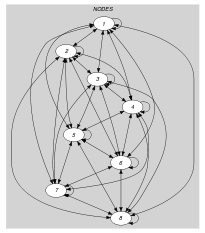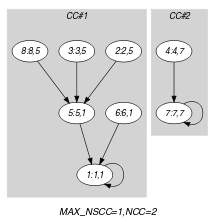(a) (b)